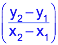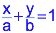## OBJECTIVE: To find the equation of a line given different conditions

Linear equations come in many forms. You've been introduced to four of them: 1. The STANDARD FORM: Ax + By = C 2. The SLOPE-INTERCEPT FORM: y = mx + b 3. The HORIZONTAL LINE: y = k 4. The VERTICAL LINE: x = k The first two are the most common and when you're asked to find the equation of a line, you're usually instructed to give your final answer in either the STANDARD FORM or the SLOPE-INTERCEPT FORM. Occasionally, you might be asked to express your final answer in: 5. The GENERAL FORM: Ax + By + C = 0, a form the line shares with conic sections. The last three linear equations shown on the foreground of the Alpine slopes above are used as starting forms depending on what is initially given in the problem. Their names are suggestive as you'll discover in a bit. 6. The POINT-SLOPE FORM: (y - y₁) = m (x - x₁), where (x₁, y₁) is a point and m is the slope. 7. The TWO-POINT FORM: (y - y₁) = ﻿(x - x₁), where (x﻿₁, y﻿₁) and (x﻿, y﻿) are two points. 8. The TWO-INTERCEPT FORM:, where a is the x-intercept and b is the y-intercept. Let's start with the POINT-SLOPE FORM. The applet below will drill you in finding the equation of a line, given a point (x₁, y₁) and the slope (m). Instructions: 1) Determine the equation of the line that has the given slope and passes through the indicated point. 2) Type your answer in either STANDARD FORM or SLOPE-INTERCEPT FORM into the input box provided. (Be sure to type EXACT VALUES of parameters!) 3) If your equation is correct, you'll see a big "CORRECT!!!" sign appear. The correct answer will also be displayed in SLOPE-INTERCEPT FORM. 4) Click the "New Problem" button to generate a new problem. Repeat at least 10 times.
Now let's move on to the TWO-POINT FORM. The applet below will drill you in finding the equation of a line, given two points (x﻿₁, y﻿₁) and (x﻿, y﻿). The applet generates only problems where the y-intercept is an integer. Instructions: 1) Determine the equation of the line that passes through the indicated points. 2) Type your answer in either STANDARD FORM or SLOPE-INTERCEPT FORM into the input box provided. (Be sure to type EXACT VALUES of parameters!) 3) If your equation is correct, you'll see a big "CORRECT!!!" sign appear. The correct answer will also be displayed in SLOPE-INTERCEPT FORM. 4) Click the "New Problem" button to generate a new problem. Repeat at least 10 times.
Finally, we're down to the last form, the TWO-INTERCEPT FORM. The applet below will drill you in finding the equation of a line, given both intercepts, the x-intercept (a, 0) and the y-intercept (0, b). Instructions: 1) Determine the equation of the line that passes through the two intercepts. 2) Type your answer in either STANDARD FORM or SLOPE-INTERCEPT FORM into the input box provided. (Be sure to type EXACT VALUES of parameters!) 3) If your equation is correct, you'll see a big "CORRECT!!!" sign appear. The correct answer will also be displayed in SLOPE-INTERCEPT FORM. 4) Click the "New Problem" button to generate a new problem. Repeat at least 10 times.

## SO there! You're now a pro at finding the equation of a line given different conditions.

Just to wrap up this session, what's your biggest takeaway in today's class?